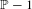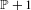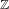Hostname: page-component-797576ffbb-bqjwj Total loading time: 0 Render date: 2023-12-05T13:47:18.625Z Has data issue: false Feature Flags: { "corePageComponentGetUserInfoFromSharedSession": true, "coreDisableEcommerce": false, "useRatesEcommerce": true } hasContentIssue false

# Closest integer polynomial multiple recurrence along shifted primes

Published online by Cambridge University Press:  20 September 2016

## Abstract

Following an approach presented by Frantzikinakis et al [The polynomial multidimensional Szemerédi theorem along shifted primes. Israel J. Math.194(1) (2013), 331–348], we show that the parameters in the multidimensional Szemerédi theorem for closest integer polynomials have non-empty intersection with the set of shifted primes$\mathbb{P}-1$ (or, similarly, of$\mathbb{P}+1$). Using the Furstenberg correspondence principle, we show this result by recasting it as a polynomial multiple recurrence result in measure ergodic theory. Furthermore, we obtain integer part polynomial convergence results by the same method, which is a transference principle that enables one to deduce results for$\mathbb{Z}$-actions from results for flows. We also give some applications of our approach to Gowers uniform sets.

Type
Original Article
Information
Ergodic Theory and Dynamical Systems , April 2018 , pp. 666 - 685

## Access options

Get access to the full version of this content by using one of the access options below. (Log in options will check for institutional or personal access. Content may require purchase if you do not have access.)

## References

Bergelson, V.. Weakly mixing PET. Ergod. Th. & Dynam. Sys. 7(3) (1987), 337349.Google Scholar
Bergelson, V. and Håland, I. J.. Sets of recurrence and generalized polynomials. Convergence in Ergodic Theory and Probability (Columbus, OH, 1993) (Ohio State University Mathematical Research Institute Publications) . de Gruyter, Berlin, 1996, vol. 5, pp. 91110.Google Scholar
Bergelson, V., Host, B., McCutcheon, R. and Parreau, F.. Aspects of uniformity in recurrence. Colloq. Math. 84/85(2) (2000), 549576.Google Scholar
Bergelson, V. and Leibman, A.. Polynomial extensions of van der Waerden’s and Szemerédi’s theorems. J. Amer. Math. Soc. 9 (1996), 725753.Google Scholar
Bergelson, V., Leibman, A. and Ziegler, T.. The shifted primes and the multidimensional Szemerédi and polynomial van der Waerden theorems. C. R. Math. Acad. Sci. Paris. 349(3–4) (2011), 123125.Google Scholar
Boshernitzan, M., Jones, R. L. and Wierdl, M.. Integer and fractional parts of good averaging sequences in ergodic theory. Convergence in Ergodic Theory and Probability. Eds. Bergelson, V., March, P. and Rosenblatt, J.. de Gruyter, Berlin, 1996, pp. 117132.Google Scholar
Frantzikinakis, N.. Multiple correlation sequences and nilsequences. Invent. Math. 202(2) (2015), 875892.Google Scholar
Frantzikinakis, N. and Host, B.. Multiple ergodic theorems for arithmetic sets. Trans. Amer. Math. Soc., to appear.Google Scholar
Frantzikinakis, N., Host, B. and Kra, B.. Multiple recurrence and convergence for sequences related to the prime numbers. J. Reine Angew. Math. 611 (2007), 131144.Google Scholar
Frantzikinakis, N., Host, B. and Kra, B.. The polynomial multidimensional Szemerédi theorem along shifted primes. Israel J. Math. 194(1) (2013), 331348.Google Scholar
Furstenberg, H.. Ergodic behaviour of diagonal measures and a theorem of Szemerédi on arithmetic progressions. J. Anal. Math. 71 (1977), 204256.Google Scholar
Gowers, W.. A new proof of Szemerédi’s theorem. Geom. Funct. Anal. 11 (2001), 465588.Google Scholar
Green, B. and Tao, T.. Linear equations in primes. Ann. of Math. (2) 171 (2010), 17531850.Google Scholar
Green, B. and Tao, T.. The Möbius function is strongly orthogonal to nilsequences. Ann. of Math. (2) 175 (2012), 541566.Google Scholar
Green, B., Tao, T. and Ziegler, T.. An inverse theorem for the Gowers U s+1[N]-norm. Ann. of Math. (2) 176(2) (2012), 12311372.Google Scholar
Koutsogiannis, A.. Integer part correlation sequences. Preprint, 2015, arXiv:1512.01313.Google Scholar
Lesigne, E.. On the sequence of integer parts of a good sequence for the ergodic theorem. Comment. Math. Univ. Carolin. 36(4) (1995), 737743.Google Scholar
Sárközy, A.. On difference sets of sequences of integers, III. Acta Math. Acad. Sci. Hungar. 31 (1978), 355386.Google Scholar
Sun, W.. Multiple recurrence and convergence for certain averages along shifted primes. Ergod. Th. & Dynam. Sys. 35 (2014), 15921609.Google Scholar
Walsh, M.. Norm convergence of nilpotent ergodic averages. Ann. of Math. (2) 175(3) (2012), 16671688.Google Scholar
Wierdl, M.. Personal communication, 2015.Google Scholar
Wooley, T. and Ziegler, T.. Multiple recurrence and convergence along the primes. Amer. J. Math. 134(6) (2012), 17051732.Google Scholar